The potential difference across 8 ohms resistance is 48 volts as shown in the figure. The value of potential difference across X and Y points will be :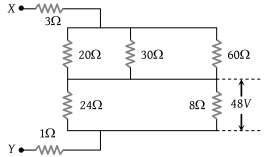(1) 160 volt

(2) 128 volt

(3) 80 volt

(4) 62 volt

Concept Questions :-

Combination of resistors
High Yielding Test Series + Question Bank - NEET 2020

Difficulty Level:

Two resistances R1 and R2 are made of different materials. The temperature coefficient of the material of R1 is α and of the material of R2 is –β. The resistance of the series combination of R1 and R2 will not change with temperature, if R1/ R2 equals :

(1) $\frac{\alpha }{\beta }$

(2) $\frac{\alpha +\beta }{\alpha -\beta }$

(3) $\frac{{\alpha }^{2}+{\beta }^{2}}{\alpha \beta }$

(4) $\frac{\beta }{\alpha }$

Concept Questions :-

Derivation of Ohm's law
High Yielding Test Series + Question Bank - NEET 2020

Difficulty Level:

An ionization chamber with parallel conducting plates as anode and cathode has $5×{10}^{7}$ electrons and the same number of singly-charged positive ions per cm3. The electrons are moving at 0.4 m/s. The current density from anode to cathode is $4\text{\hspace{0.17em}}\mu A/{m}^{2}$. The velocity of positive ions moving towards cathode is :

(1) 0.4 m/s

(2) 16 m/s

(3) Zero

(4) 0.1 m/s

Concept Questions :-

Current and current density
High Yielding Test Series + Question Bank - NEET 2020

Difficulty Level:

A wire of resistance 10 Ω is bent to form a circle. P and Q are points on the circumference of the circle dividing it into a quadrant and are connected to a Battery of 3 V and internal resistance 1 Ω as shown in the figure. The currents in the two parts of the circle are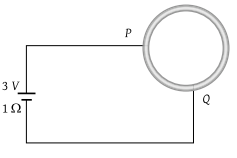(1)

(2) $\frac{5}{26}A\text{\hspace{0.17em}\hspace{0.17em}and\hspace{0.17em}}\frac{15}{26}A$

(3)

(4)

Concept Questions :-

Kirchoff's voltage law
High Yielding Test Series + Question Bank - NEET 2020

Difficulty Level:

In the given circuit, it is observed that the current I is independent of the value of the resistance R6. Then the resistance values must satisfy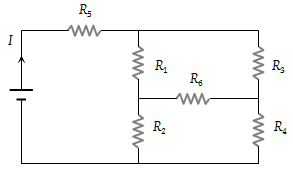(1) ${R}_{1}{R}_{2}{R}_{5}={R}_{3}{R}_{4}{R}_{6}$

(2) $\frac{1}{{R}_{5}}+\frac{1}{{R}_{6}}=\frac{1}{{R}_{1}+{R}_{2}}+\frac{1}{{R}_{3}+{R}_{4}}$

(3) ${R}_{1}{R}_{4}={R}_{2}{R}_{3}$

(4) ${R}_{1}{R}_{3}={R}_{2}{R}_{4}={R}_{5}{R}_{6}$

Concept Questions :-

Combination of resistors
High Yielding Test Series + Question Bank - NEET 2020

Difficulty Level:

In the given circuit, with a steady current, the potential drop across the capacitor must be :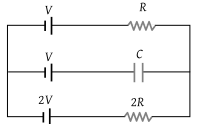(1) V

(2) V / 2

(3) V / 3

(4) 2V / 3

Concept Questions :-

Kirchoff's voltage law
High Yielding Test Series + Question Bank - NEET 2020

Difficulty Level:

A wire of length L and 3 identical cells of negligible internal resistances are connected in series. Due to current, the temperature of the wire is raised by ΔT in a time t. A number N of similar cells is now connected in series with a wire of the same material and cross–section but of length 2 L. The temperature of the wire is raised by the same amount ΔT in the same time t. the value of N is

(1) 4

(2) 6

(3) 8

(4) 9

Concept Questions :-

Grouping of cells
High Yielding Test Series + Question Bank - NEET 2020

Difficulty Level:

What is the equivalent resistance between the points A and B of the network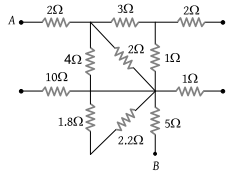(1) $\frac{57}{7}\Omega$

(2) 8 Ω

(3) 6 Ω

(4) $\frac{57}{5}\Omega$

Concept Questions :-

Combination of resistors
High Yielding Test Series + Question Bank - NEET 2020

Difficulty Level:

The effective resistance between points P and Q of the electrical circuit shown in the figure is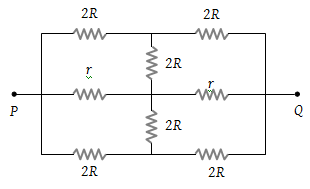(1) $2Rr/\left(R+r\right)$

(2) $8R\text{\hspace{0.17em}}\left(R+r\right)/\left(3R+r\right)$

(3) $2r+4R$

(4) $5R/2\text{\hspace{0.17em}\hspace{0.17em}}+\text{\hspace{0.17em}}2r$

Concept Questions :-

Combination of resistors
High Yielding Test Series + Question Bank - NEET 2020

Difficulty Level:

In the circuit element given here, if the potential at point B, VB = 0, then the potentials of A and D are given as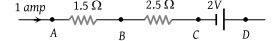(1) ${V}_{A}=-1.5\text{\hspace{0.17em}}V,\text{\hspace{0.17em}}{V}_{D}=+2\text{\hspace{0.17em}}V$

(2) ${V}_{A}=+1.5\text{\hspace{0.17em}}V,\text{\hspace{0.17em}}{V}_{D}=+2\text{\hspace{0.17em}}V$

(3) ${V}_{A}=+1.5\text{\hspace{0.17em}}V,\text{\hspace{0.17em}}{V}_{D}=+0.5\text{\hspace{0.17em}}V$

(4) ${V}_{A}=+1.5\text{\hspace{0.17em}}V,\text{\hspace{0.17em}}{V}_{D}=-0.5\text{\hspace{0.17em}}V$

Concept Questions :-

Kirchoff's voltage law